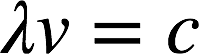# Problem: Cesium was discovered in natural mineral waters in 1860 by R. W. Bunsen and G. R. Kirchhoff, using the spectroscope they invented in 1859. The name came from the Latin caesius (“sky blue”) because of the prominent blue line observed for this element at 455.5 nm. Calculate the frequency and energy of a photon of this light.

###### FREE Expert Solution

Recall: The energy of a photon is given by the equation:where h = Planck's constant and v = frequency of light. Frequency and wavelength (λ) are related via the following equation:where c = speed of light (3.00 x 108 m/s).

96% (314 ratings)###### Problem Details

Cesium was discovered in natural mineral waters in 1860 by R. W. Bunsen and G. R. Kirchhoff, using the spectroscope they invented in 1859. The name came from the Latin caesius (“sky blue”) because of the prominent blue line observed for this element at 455.5 nm. Calculate the frequency and energy of a photon of this light.

Frequently Asked Questions

What scientific concept do you need to know in order to solve this problem?

Our tutors have indicated that to solve this problem you will need to apply the The Energy of Light concept. You can view video lessons to learn The Energy of Light. Or if you need more The Energy of Light practice, you can also practice The Energy of Light practice problems.

What professor is this problem relevant for?

Based on our data, we think this problem is relevant for Professor Arasasingham's class at UCI.

What textbook is this problem found in?

Our data indicates that this problem or a close variation was asked in Chemistry: An Atoms First Approach - Zumdahl Atoms 1st 2nd Edition. You can also practice Chemistry: An Atoms First Approach - Zumdahl Atoms 1st 2nd Edition practice problems.